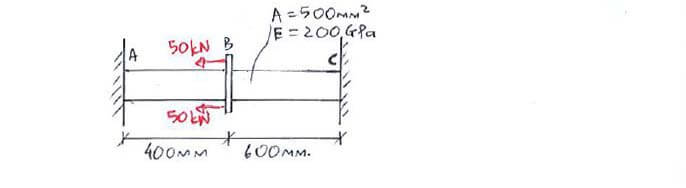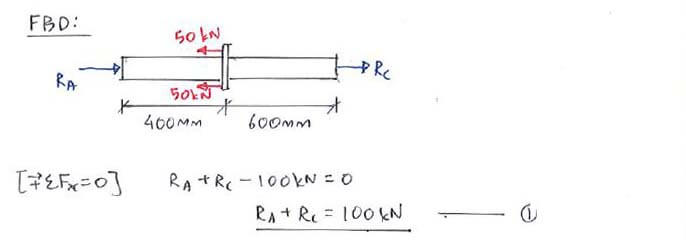# Example QuestionClick below to show answer. X

#### Step 1: 1st equation from [ΣFx = 0] Step 1: 1st equation from [ΣFx = 0]#### Useful formula: Useful formula:Now that you've mastered this question, you can try Question 1.